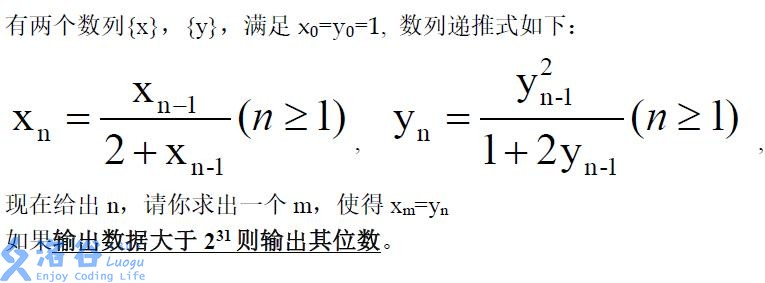2019-02-16 16:02:17

## 题目大意## 数据范围

n<=maxlongint

### 做法

#include<cstdio>
#include<cstring>
#include<iostream>
#include<algorithm>
using namespace std;
int n,cnt=0;
double a=2.0;
void pow(int k)
{
if(k<=1) return;
pow(k/2);
a=a*a;cnt*=2;
while(a>10) a/=10,cnt++;
if(k%2==1)
{
a*=2;
if(a>10) a/=10,cnt++;
}
}
int main()
{
scanf("%d",&n);
if(n<=31)
{
int res=1;
for(int i=1;i<=n;i++)
res=res*2;
printf("%d",res-1);
}
else
{
pow(n);
printf("%d",cnt);
}
return 0;
}
• star
首页